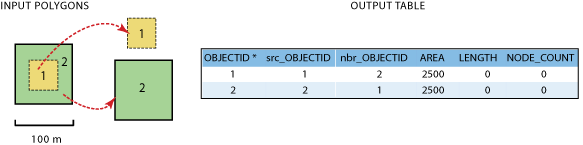# How Polygon Neighbors Works

How the ArcGIS geoprocessing tool Polygon Neighbors finds neighbors and populates the output table.

## Details on how neighboring relationships are found and statistics are calculated

The neighboring relationships are defined as follows:

• Overlapping neighbors—polygons that have all or part of their areas overlapping
• Edge neighbors—polygons that have common or touching boundaries
• Node neighbors—polygons that touch at a point (boundary crossing or touching)

Neighboring polygons are found according to the same rules for polygons as the Intersect tool.

##### Note:

The following discussion assumes use of the tool dialog box. See the Polygon Neighbors tool documentation for scripting syntax.

The Polygon Neighbors tool follows a hierarchical path to determine the type of neighbor and the statistics to record in the output table. The neighboring relationships, in hierarchical order from high to low, are overlapping, coincident edge, and node neighbors. Once a higher-order neighbor is found, the tool calculates and stores the relationship information and the analysis of lower-order relationships is skipped. The detailed workflow is as follows:

• Select a polygon to use as the source feature.
• Find all the polygons that intersect the source polygon (find the neighbors).
• For the first neighbor found, analyze the following for the source polygon:
• If the neighbor polygon is an overlapping neighbor and the Include area overlaps check box is checked
• Add the AREA field to the output table.
• Calculate the area of the overlap.
• Record the calculated area for use in the output table AREA field.
• Record 0 for use in the output table LENGTH field.
• Record 0 for use in the output table NODE_COUNT field.
• Overlapping neighbor analysis is complete. Analyze the next neighbor polygon.
• If the neighbor polygon is an edge neighbor
• Calculate the length of the coincident boundary.
• Record the calculated length for use in the LENGTH field.
• Record 0 for use in the NODE_COUNT field.
• Edge neighbor analysis is complete. Analyze the next neighbor polygon.
• If the neighbor polygon is a node neighbor
• Find the number of times the neighbor polygon crosses and touches the source polygon at a point.
• Record this count value for use in the NODE_COUNT field.
• Record 0 for use in the LENGTH field.
• Node neighbor analysis is complete. Analyze the next neighbor polygon.

See below for examples of the above process.

Once the neighboring relationships have been found, as described above, the tool uses the Report By Field(s) in order to determine how to report the neighbor relationships and statistics in the output table. The Report By Field(s) parameter is used to identify unique polygons or polygon groups and report their neighboring information by the unique polygons or polygon groups. Polygons of the same group have the same set of field values.

### Report By Field(s) Example 1—Using a field with unique values for every individual polygon

When you need to find the neighbors of each individual polygon in the input, specify an input field that has a unique value for every polygon. In this example, the input table of the nine polygons has a field myCode, which has a unique value for every individual polygon.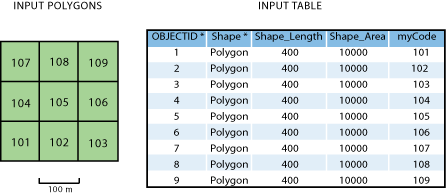The table below shows the result of using the myCode field as the Report By Field(s). Notice the prefixes in the source and neighbor field names: src_myCode and nbr_myCode.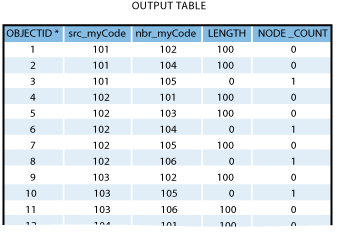### Report By Field(s) Example 2—Using a field with values defining unique polygon groups

When you need to identify unique polygon groups and report the neighboring information by the groups, you can specify a field that carries unique values of the classification. The neighboring information is summarized based on how the unique groups are related. In this example, the unique polygon groups are identified by using the input field myClass as the Report By Field(s).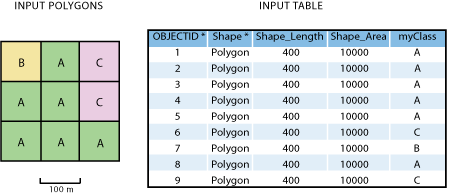Below are the results of using myClass as the Report By Field(s):

• For group A to other polygons of group A, the total length of coincident edges is 1200, which comes from the six coincident edges among the polygons in group A.
##### Note:

The output table will only contain a unique record for each unique combination of source and neighbor polygon values used in the Report By Field(s). Since group A is used as the source and the neighbor as one side of the relationship, and the reversed relationship is also group A as the source and the neighbor, the total of both sides of the neighbor relationship are in one record.

• Between group A and group B, there are only two edges that are coincident for a total length of 200. This is also the case between group B and group A.
• Between group A and group C, there are three coincident edges for a total length of 300. This is also the case between group C and group A.
• For group B to other polygons of group B, unlike group A, there is no neighboring relationship found; therefore, there is nothing reported in the output.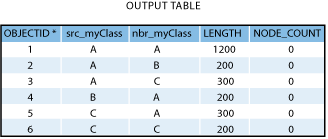### Report By Field(s) Example 3—Using multiple fields with combined values defining unique polygon groups

In this example, the values in the field myZone represent one classification of the polygons; the values in the field myClass represent another classification.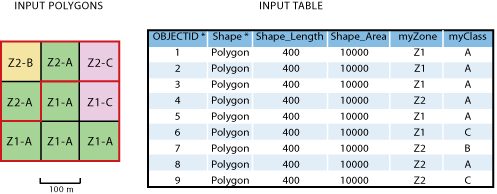When you specify two fields, myZone and myClass, as the Report By Field(s), the combined values of the two fields will result in the following unique polygon groups:

• Z1-A group
• Z1-C group
• Z2-A group
• Z2-B group
• Z2-C group

The output table below shows the neighboring information among the above groups. The first eight rows represent the unique groups of Z1 values combined with the values in the field myClass being the source groups and their neighboring groups in Z1 and Z2. In particular:

• The Z1-A source group has six coincident edges within the group itself (similar to the group A in the previous example); therefore, the total length of coincident edges is 600.
• The Z1-A source group is an edge neighbor with the Z1-C neighbor group; the total length of coincident edges is 200.
• The Z1-A source group is an edge neighbor with the Z2-A neighbor group; the total length of coincident edges is 300.
• The Z1-A source group is not an edge neighbor with the Z1-B neighbor group, but Z1-B neighbor group boundary does touch the Z1-A source group at a node and is a node neighbor. Therefore, the value for LENGTH is 0, and the value for NODE_COUNT is 1.
• You can use the same logic to interpret the rest of the information.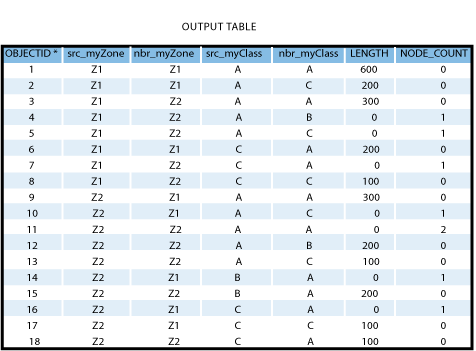## Running PolygonNeighbors against input data with and without overlapping polygons

The following examples show details of how the neighboring relationships are analyzed in hierarchical order from high to low when running against input data with and without area overlap. All the examples below use a single field as the Report By Field(s) which contains unique values for each polygon.

### Example 1—Input data containing no overlapping polygons

The input data used in the following two cases contain nonoverlapping polygons.

#### a. Include area overlap check box unchecked (default)

The four input polygons shown below are not overlapping. When the tool is run with the Include area overlap check box unchecked, the tool will only look for edge and node neighbors, in that order. Using polygon 1 as the source polygon for an example, three neighbor polygons are found, and the following information is reported in the output table below:

• Polygon 2 has a coincident edge with polygon 1; therefore, it is an edge neighbor. The length of the coincident edge, 100, is written in the LENGTH field. The node neighbor analysis is skipped, and the field NODE_COUNT gets a value of 0. This is the case for polygon 4 as well.
• Polygon 5 does not have a coincident edge with polygon 1; the field LENGTH gets a value of 0. However, polygon 5 does touch polygon 1 at one point; therefore, it is a node neighbor of polygon 1 and gets the value of 1 for the NODE_COUNT field.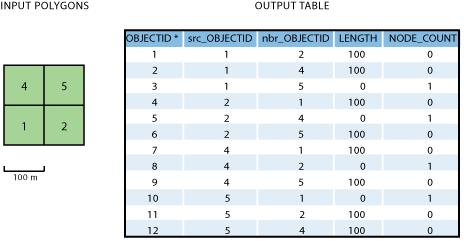#### b. Include area overlap check box checked

In this case, the output table will contain the field AREA. All of the AREA field values will be 0 since none of the polygons overlap. The analysis of edge and node neighbors continues, and you get the same values in the LENGTH and NODE_COUNT fields as you did in Example 1-a above.

### Example 2—Input data containing overlapping polygons

The input data used in the following two cases contains overlapping polygons.

#### a. Include area overlap check box unchecked (default)

Among the following four polygons, overlapping, edge, or node neighbors occur only once between any two polygons. The tool only looks for edge and node neighbors (in that order) and the output does not contain the field AREA. Using polygon 1 as the source polygon for an example, three neighbor polygons are found, and the following information is reported in the output table below:

• Each of polygons 2 and 5 has a coincident edge with polygon 1; the length of the edge, 20, is written in the LENGTH field. The node neighbor analysis is skipped even though polygon 2 crosses polygon 1 at a point. The field NODE_COUNT gets a value of 0.
• Polygon 4 has a coincident edge with polygon 1; the length of the coincident edge, 100, is written in the LENGTH field.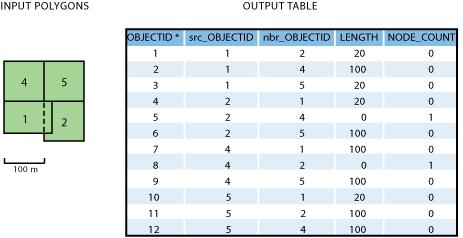#### b. Include area overlap check box checked

Using polygon 1 as the source polygon for an example, three neighbor polygons are found, and the following information is reported in the output table below:

• Polygon 2 overlaps polygon 1; the area of overlap, 1600, is written in the AREA field. The analysis of edge and node neighbors is skipped even though polygon 2 has a coincident edge with polygon 1 and crosses it at a point. Therefore, the fields LENGTH and NODE_COUNT get a value of 0.
• Polygon 4 has a coincident edge with polygon 1; the coincident length of the edge, 100, is written in the LENGTH field.
• Polygon 5 has a coincident edge with polygon 1; the length of the coincident edge, 20, is written in the LENGTH field. The node neighbor analysis is skipped even though it crosses polygon 1 at a point. The field NODE_COUNT gets a value of 0.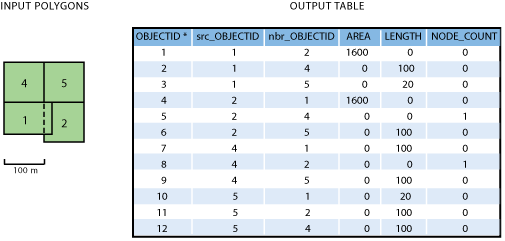### Example 3—Input data containing polygons that intersect another polygon more than once

In the input data used in the following cases, overlapping, edge, or node neighbors occur more than once between two polygons. The values in the output fields—AREA, LENGTH, and NODE_COUNT—are the summations of all occurrences in each neighbor type.

#### a. Include area overlap check box unchecked (default)

The two polygons below overlap in two places and have two coincident edges. The tool does not analyze the overlap between the two features. It finds the two coincident edges and writes the summation of the two lengths of the coincident edges, 40, to the LENGTH field. The analysis of node neighbors is skipped, so a value of 0 is entered into the NODE_COUNT field. This is the case for polygon 1 being the source polygon and polygon 2 being the neighbor, and vice versa.

#### b. Include area overlap check box checked

For the same two polygons below, the tool finds two area overlaps and writes the summation of the two areas, 800, in the AREA field. The analysis for edge and node neighbors is skipped. Therefore, a value of 0 is entered in the LENGTH and NODE_COUNT fields. This is the case for polygon 1 being the source polygon and polygon 2 being the neighbor, and vice versa.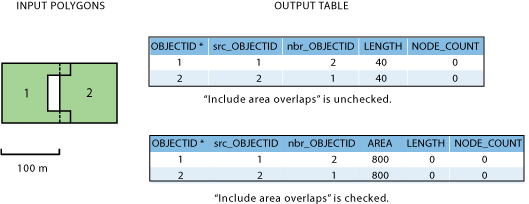#### c. Include area overlap check box unchecked (default) and no coincident edges exist between the input polygons

The tool finds no coincident edges and writes 0 to the LENGTH field. It continues with the analysis of node neighbors and finds that the boundaries cross twice. Therefore, it writes the value 2 in the NODE_COUNT field. This is the case for polygon 1 being the source polygon and polygon 2 being the neighbor, and vice versa, as shown in the output table below: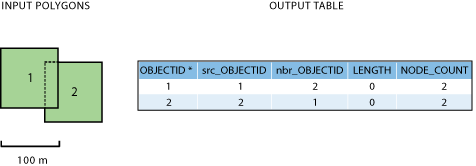### Example 4—Special Cases

The input data used in the next two special cases may seem the same but cover the area differently as explained below.

#### a. A polygon covering the hole of another polygon

In this case, polygon 2 has a hole which is covered by polygon 1. Notice the two polygons do not overlap, so the analysis for overlapping neighbors is unnecessary. When polygon 1 is the source polygon and polygon 2 has a coincident edge of 200 with polygon 1, this value is written to the LENGTH field. The NODE_COUNT field value is 0. Similarly, when polygon 2 is the source polygon, polygon 1 has a coincident edge of 200 with polygon 2, which results in the same values in the LENGTH field for each record.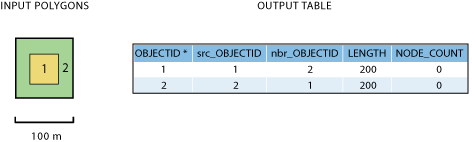#### b. A polygon completely overlapping another polygon

In this case, polygons 1 and 2 overlap each other. You need to check the Include area overlap check box in order for this neighboring relationship to be found in the analysis. For both polygon 1, being the source, and polygon 2, being the neighbor, and vice versa, the overlapping area of 2500 is reported in the output table below.